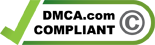# Numerical Test

The numerical reasoning test is a type of psychometric test which is very popular among test publishers and often used in recruitment processes. The numerical exams aim to assess the candidate's ability to analyze mathematical problems and draw logical conclusions in a limited time. There are two types of numerical aptitude tests: the numeracy test and the numerical reasoning test. You can learn more about these aptitude tests by consulting our guides below.

## Numerical Reasoning Test

The numerical reasoning test highlights the candidate's ability to quickly grasp numerical concepts, solve mathematical problems and make sound and logical decisions, using numbers and any other given information.

## Basic Numeracy Test

The numeracy test is the most basic numerical aptitude test and is designed to assess the testee's ability to manipulate basic mathematical concepts without the help of a calculator. This cognitive test assesses the candidate's ability to analyze mathematical problems and draw logical conclusions in a limited time.